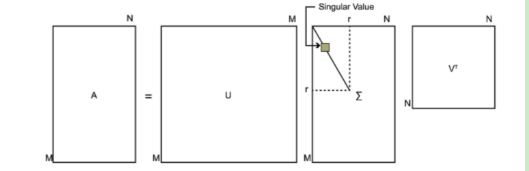# 奇异值分解

Posted by MaggicQ on September 18, 2018

## 奇异值分解

### SVD的数学含义

SVD同样是对矩阵进行分解，但不要求矩阵$A$一定是方阵，假设矩阵$A = A_{m \times n}$ ，那么容易得到，$AA^T$的大小为$m \times m$的实对称方阵，$A^TA$的大小为$n \times n$的实对称方阵， 由上述可知，实对称方阵存在对称对角化分解，假设 $AA^T = P\Lambda_1 P ^T, A^TA = Q\Lambda_2Q^T$，则矩阵A的奇异值分解为

SVD示意图：